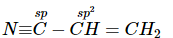Courses

# SRMJEEE Chemistry Mock Test - 2

## 35 Questions MCQ Test SRMJEEE Subject Wise & Full Length Mock Tests | SRMJEEE Chemistry Mock Test - 2

Description
This mock test of SRMJEEE Chemistry Mock Test - 2 for JEE helps you for every JEE entrance exam. This contains 35 Multiple Choice Questions for JEE SRMJEEE Chemistry Mock Test - 2 (mcq) to study with solutions a complete question bank. The solved questions answers in this SRMJEEE Chemistry Mock Test - 2 quiz give you a good mix of easy questions and tough questions. JEE students definitely take this SRMJEEE Chemistry Mock Test - 2 exercise for a better result in the exam. You can find other SRMJEEE Chemistry Mock Test - 2 extra questions, long questions & short questions for JEE on EduRev as well by searching above.
QUESTION: 1

### With oxalic acid, glycerol at 503 K gives

Solution: When glycerol is reacted with Oxalic acid at 383K, it gives formic acid as follows: CH2OH-CHOH-CH2OH + COOH-COOH ------> CH2OH-CHOH-CH2OH + HCOOH at 383K . The glycerine is used in excess amounts and hence obtained in products also. But when , it is heated with Oxalic acid at 503K, it gives allyl alcohol, i.e. those alcohols in which OH group attached to carbon is sp3 hybridised but the sp3 hybridised carbon is attached to sp2 hybridised carbon atom.Allyl alcohol is CH2=CH-CH2OH.
QUESTION: 2

Solution:
QUESTION: 3

### The reaction of chloroform with alcoholic KOH and p-toluidine form

Solution:
QUESTION: 4

The acidic solution of salt produced a deep blue colour with starch iodide solution. The salt may be

Solution:
QUESTION: 5

Benzene is obtained by fractional distillation of

Solution:
QUESTION: 6

The quantum number values for the designation 3d are

Solution:
QUESTION: 7

Other factors being constant which bond order is expected to correspond to shortest bond length

Solution:
QUESTION: 8

The most stable carbonium ion is:

Solution:
QUESTION: 9

A carboxylic acid is converted into its anhydride using

Solution:
QUESTION: 10

Carbohydrates are used by body mainly

Solution:
QUESTION: 11

The bond between carbon - 1 and carbon -2 in the compound N ≡ C − CH = CH2 is

Solution:QUESTION: 12

When enthalpy and entropy change for a chemical reaction are -2.5 x103 cals and 7.4 cals deg⁻1 respectively . Predict that reaction at 298 K is

Solution:
QUESTION: 13

The chemical equilibrium of a reversible reaction is not influenced by

Solution:
QUESTION: 14

The rate of reaction, A + B → products; is given by the equation, r = k [A] [B]. If B is taken in large excess, the order of the reaction would be

Solution:
QUESTION: 15

Streptomycin, a well known antibiotic, is a derivative of

Solution:
QUESTION: 16

Which of the following electronic configuration corresponds to an inert gas?

Solution:

As the electronic configuration of inert gases is ns2 np6 ,thus the element containing electronic configuration 1s22s22p6 , is an inert gas.

QUESTION: 17

The IUPAC name of the compound having the molecular formula Cl₃C-CH₂CHO is

Solution:
QUESTION: 18

The heat of combustion of methane at 298K is expressed by CH4 (g) + 2O2 (g) → CO2 (g) + 2H2O (l), ΔH = − 890.2 k J. The magnitude of ΔE of the reaction at this temperature is

Solution:
QUESTION: 19

The limiting molar conductivities for NaCl. KBr and KCl are 126, 152 and 150 S-cm2-mol⁻1 respectively. The molar conductivity of NaBr is

Solution:
QUESTION: 20

Oxygen and cyclopropane at partial pressure of 570 torr and 170 torr respectively are mixed in a gas cylinder. What is the ratio of the number of moles of cyclopropane to the number of moles of oxygen ?

Solution:
QUESTION: 21

Which of the following compound is used to prevent the deposition of oxides of lead on spark plug, combustion chamber and exhaust pipe?

Solution:
QUESTION: 22

When HCl gas is passed through a saturated Solutions of NaCl , the solubility of Nacl:

Solution:
QUESTION: 23

Which of the following molecule having molecular formula C₄H₆O₂ will be optically active?

Solution:
QUESTION: 24

1 g of radioactive element reduces to 125 mg after 24 hours. Half life of isotope is

Solution:
QUESTION: 25

Which one of the following is a typical example of compound which exists as zwitter ion?

Solution:
QUESTION: 26

A substance C₄H₁₀O yields on oxidation a compound C₄H₈O, which gives an oxime and a positive iodoform test. The original substance on treatment with conc. H₂SO₄ gives C₄H₈. The structure of the compound is

Solution:
QUESTION: 27

Select incorrect statements(s):

Solution:
QUESTION: 28

Which of the following is an example of natural polymer?

Solution:
QUESTION: 29

If 0.6387 of plantinichloride of a monoacid base on ignition gave 0.209g of platinum, then molecular weight of the base is

Solution:
QUESTION: 30

Which acts as both oxidising as well as reducing agent ?

Solution: As here in B we can see that N is in +3oxidation state therefore it can increase up to 5 or decrease down to -3 hence it will oxidise as well as reduce.
QUESTION: 31

In a metal 'M' having BCC arrangement, edge length of the unit cell is 400 pm. The atomic radius of M is

Solution:
QUESTION: 32

The volume of 0.6 M NaOH required to neutralize 30 cm3 of 0.4 M HCI is

Solution:
QUESTION: 33

The packing fraction for a body-centred cubic is

Solution:
QUESTION: 34

A chemical reaction should

Solution:
QUESTION: 35

If dispersed phase is a liquid and the dispersion medium is a solid, the colloid is known as

Solution: